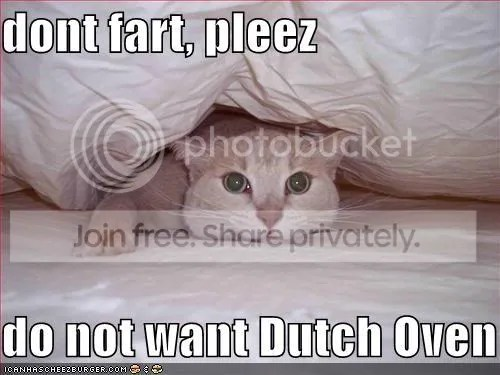# Unit 10: Gas Laws OverviewToday we’re going to begin studying Gas Laws… (and that doesn’t mean the rules on Dutch Oven or letting one go in a crowded elevator).

We’re going to try some new classroom techniques for this final unit and create an “Open” classroom. Below are the expectations of you the student and of me the teacher.

# Unit 10: Complete the Puzzle

## 6th Period Chemistry – Activity Chart

Gas Laws Resource Living Document

### 1. Properties of Gases

• Describe and site 4 examples of how gases are different than solids and liquids at the molecular level.
• Complete each of these conversions:   atm → mmHg   //   atm → kPa   //   atm → torr

### 2. Boyle’s Law

• Describe Boyle’s Law
• Complete 4 problems using Boyle’s law
• Write out an explanation of how you can remember the formula for Boyle’s Law.

### 3. Charles Law

• Describe Charles law
• Complete 4 problems using Charles’ law
• Watch this video and write an explanation of how it proves Charles’ Law.

### 4. Gay-Lussac’s Law

• Describe Gay-Lussac’s law
• Before receiving completion for this section you should  have: Posted at least 1 source of your  information (that was not previously posted) , with your name to the Google DocORPost a question you have and/or Post a response to another students question.

### 5. Combined Gas Law

• Complete 4 problems using the Combined Gas Law.
• Write and submit an explanation of what the combined gas law is and why it is useful in the box below. You can see the text from other respondents by clicking this link. Combined Gas Law Responses

### 6. Standard Temperature & Pressure (STP)

• Complete 2 problems using the Combined Gas Law at STP.
• Write a Definition of Standard Temperature & Pressure.

### 7. Universal Gas Constant (R)

• Find ‘R’s value for :
• L·Torr/mol·K (L·mmHg/mol·K)
• liter·atm/mol·K
• Determine what formula is ‘R’ used in.

### 8. Ideal Gas Law: Volume

• Write out the formula of the Ideal Gas Law.
• Complete 4 problems finding the value of the Universal Gas constant using the Ideal Gas Law.

### 9. Ideal Gas Law: Moles

• Determine how many liters are equal to 1 mole of any substance at STP (#19 on the Gas Laws Calculations)
• Complete  4 problems using the Ideal Gas law.

### 10. Density

• Label the parts of this formula:  ρ = MM·P/RT
• Complete 5 problems for density of Gases.